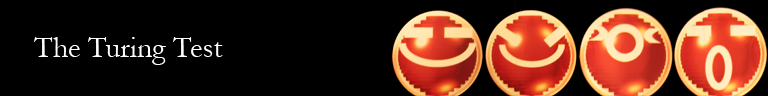##Main | Introduction | The Turing Test | The Loebner Prize | Strong AI | Sources | About
Data Processing Objection | Argument from Consciousness | Mechanical Objection | Key Flaw of the Turing Test

### Data Processing Objection

This objection states that machines process data bit-by-bit, in sequential order, whereas humans view data holistically. Therefore machines can never be truly human, since their way of thinking if structured fundamentally differently. (Turing)

Turing addresses this objection in mathematical terms, giving a situation in which a human and a computer were both asked to give the value of pi. The human would most likely give a rounded answer such as 3.14 or even 3, whereas the computer would be able to give a very precise answer. However, in the context of the test, both of these values are "correct", as they are acceptable answers of pi to an interrogator. Indeed, the computer would even be able to randomly select a rounded-off value of pi as to behave in a more human manner. Turing's conclusion is that these differences are immaterial in the context of the test. (Turing)